These grade 1 worksheets introduce students to fractions.  The focus is on gaining a conceptual understanding of what a fraction means; this is largely approached by comparing fractions to simple shapes which have been divided into equal parts.    Fractions of a group or set are also introduced.

## "Equal parts" worksheets

Example

Identifying equal parts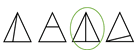Dividing shapes into equal parts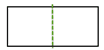## Writing fractions worksheets

Parts of a whole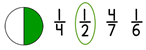Fractions in words   one half = 1/2
Coloring shapes to make fractions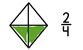Writing fractionsFractions of a group or set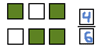## Fraction word problems

Write the fraction from the story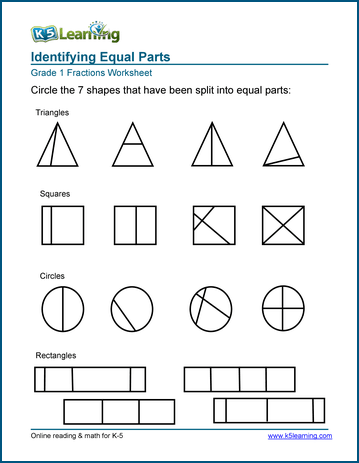Sample Grade 1 "Equal Parts" Fractions Worksheet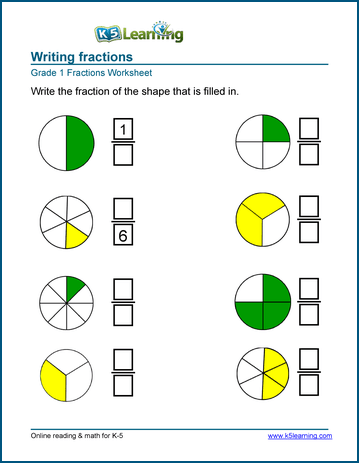Sample Grade 1 Writing Fractions Worksheet

## More fractions worksheets

Explore all of our fractions worksheets, from dividing shapes into "equal parts" to multiplying and dividing improper fractions and mixed numbers.

## What is K5?

K5 Learning offers reading and math worksheets, workbooks and an online reading and math program for kids in kindergarten to grade 5.  We help your children build good study habits and excel in school.